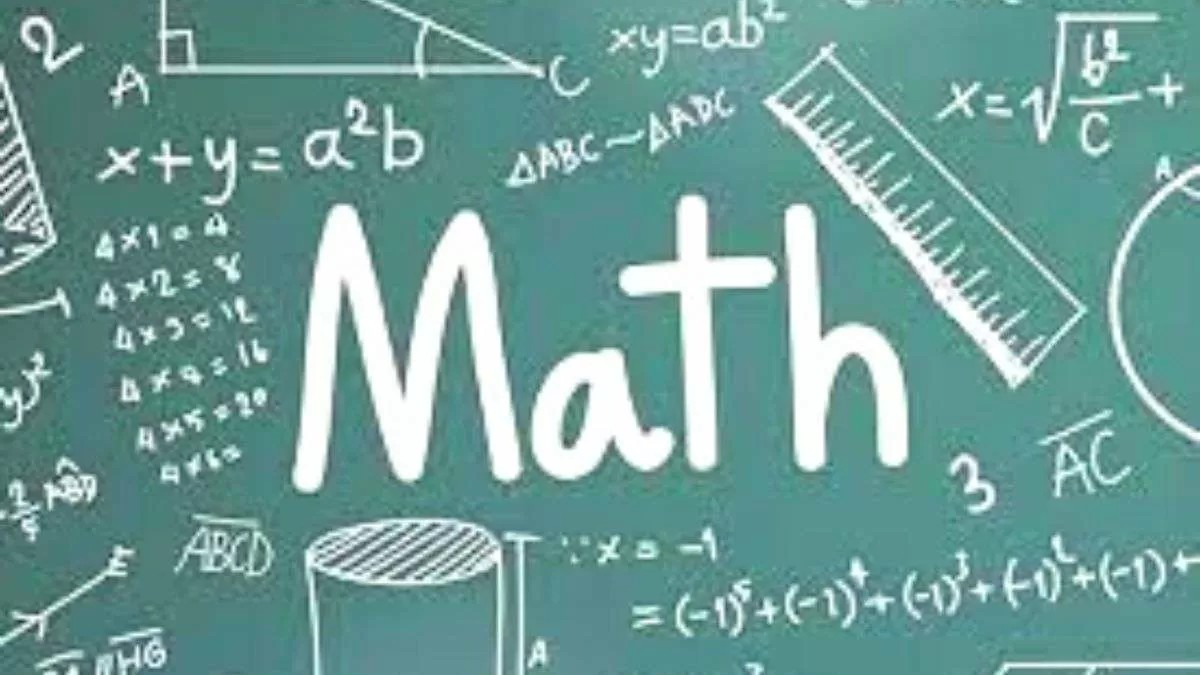# Math riddles: These math riddles are fun and easy. Try solving these!

Math is a subject that is hated by many, but in reality, it can sometimes be easy!Many people defame math for its complex nature. However, the subject can be fun, exciting, and in fact, simple too. These math riddles are proof!

Math Riddle 1:

X is a magical number. Add, subtract, or multiply it with any number, and no change will happen. Do you know “X”?

Math Riddle 2:

What do you think a math exam paper says to the other?

Math Riddle 3:

Which of the following is correct?

• Eighty-seven plus fifty-three is One hundred and thirty-nine.
• Eighty-seven plus fifty-three are One hundred and thirty-nine.

Math Riddle 1:

X is a magical number. Add, subtract, or multiply it with any number, and no change will happen. Do you know “X”?

The magical number X is 0.

The Milk Is Kept In Open! Find The Hidden Cat Before She Drinks It All In This Optical Illusion Image!

Math Riddle 2:

What do you think a math exam paper says to the other?

The math exam paper says “I have a lot of problems!”

Math Riddle 3:

Which of the following is correct?

• Eighty-seven plus fifty-three is One hundred and thirty-nine.
• Eighty-seven plus fifty-three are One hundred and thirty-nine.